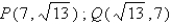# Algebra and Trigonometry

Mathematics

## Quiz 1 : Functions and Their GraphsStudy FlashcardsLooking for Calculus Homework Help?

## Quiz 1 :Functions and Their Graphs

Question TypeGraph the function.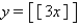Free
Multiple Choice

C

Tags
Choose question tagFind the midpoint of the line segment PQ. P(10, 1), Q(4, 7)
Free
Multiple Choice

A

Tags
Choose question tagTell where the function is increasing.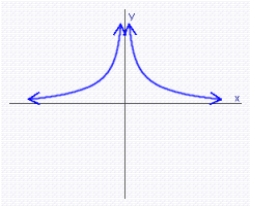Free
Multiple Choice

A

Tags
Choose question tagLet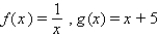. Find the composite function which expresses the given correspondence correctly.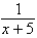Multiple Choice
Tags
Choose question tagFind the graph of the equation. f (x) = | x - 4 |
Multiple Choice
Tags
Choose question tagLet f (x) = 2x + 1, g(x) = 3x - 2. Find the function.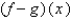Multiple Choice
Tags
Choose question tagLet f (x) = 2x - 1, g(x) = 3x - 2. Find the domain of the function.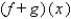Multiple Choice
Tags
Choose question tagGraph the piecewise-defined function.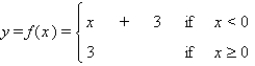Multiple Choice
Tags
Choose question tagFind the midpoint between the two points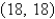and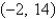.
Multiple Choice
Tags
Choose question tagFind the slope of the line through P(-1,4) and Q(-5,0).
Multiple Choice
Tags
Choose question tagDraw the line using the slope and y-intercept. y + 2 = 3x
Multiple Choice
Tags
Choose question tagFind the distance between the two points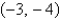and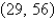.
Multiple Choice
Tags
Choose question tagFind the slope of the line determined by the equation.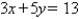Multiple Choice
Tags
Choose question tagFind the slope of the line passing through the pair of points: P (-19,-5); Q (-9,6)
Multiple Choice
Tags
Choose question tagWrite the equation of the line that passes through the point P (1, 4) and is perpendicular to the line y = - 4x + 18.
Multiple Choice
Tags
Choose question tagEvaluate the difference quotient for the function. f (x) = 2x - 3
Multiple Choice
Tags
Choose question tagWhich graph represents the function? g(x) =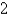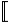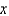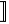Multiple Choice
Tags
Choose question tagFind the graph of the equation.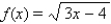Multiple Choice
Tags
Choose question tagFind the slope of the line.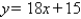Multiple ChoiceFind the slope of the line passing through the pair of points.